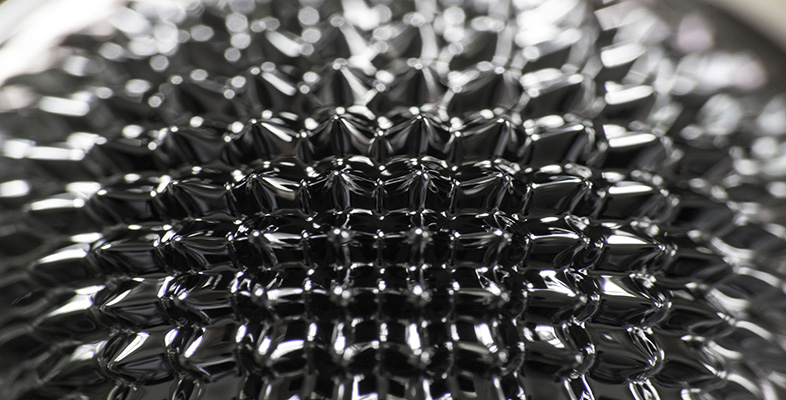James Clerk Maxwell

This free course is available to start right now. Review the full course description and key learning outcomes and create an account and enrol if you want a free statement of participation.

Free course

# 3.2 Generalising Ampère's law

We need to generalise Ampère's law beyond the confines of static charge densities. Let's try adding an extra (and at this stage unknown) vector field, K to the right-hand side of the differential form of Ampère's law. The modified equation then readsWhat can be said about the term K? Taking the divergence of both sides of the modified equation, and using the fact that the divergence of any curl is equal to zero, we obtainSo, using the equation of continuity (Equation 7.4), we haveNow Gauss's law tells us thatso, using Equation 7.7 to interchange the order of the time and space derivatives,We conclude thatThe simplest solution to this equation isbut there are other solutions as well. In fact, the most general solution iswhere X is any smooth vector field. You can easily verify that this satisfies Equation 7.8, because taking the divergence of both sides givesand the last term vanishes, being the divergence of a curl.

This is as far as mathematical analysis can take us. It is not too surprising that we still have a choice to make. You should not expect to derive a fundamental law of physics from other laws. As a general rule, however, it is sensible to adopt the simplest law that is consistent with all the known facts. That is what Maxwell did, and we shall follow his lead. We assume that curl X = 0 in Equation 7.9, and replace Ampère's law byThis equation is called the Ampère–Maxwell law and the additional term, ε0μ0E/∂t, is called the Maxwell term. Some authors refer to the Maxwell term as μ0 times the displacement current density. We will not use this terminology in this course, but an optional appendix (best read after this section) describes the curious logic behind it (see Section 6). The Ampère–Maxwell law is the last of Maxwell's four equations of electromagnetism. It is believed to be true in all situations, both static and dynamic.

The above argument for the Ampère–Maxwell law is driven by theory. If we believe the law of conservation of charge (as we do), our mathematical analysis shows that Ampère's law must be modified for time-dependent situations. The simplest modification, consistent with Gauss's law and the equation of continuity, is then given by the Ampère–Maxwell law. Physics walks forward on the two legs of theory and experiment. Sometimes experiment strides ahead and reveals new facts which cry out for theoretical interpretation. The Ampère–Maxwell law is an early example of the opposite process – a law that emerged from a theoretical argument, and cried out for experimental confirmation.

In Maxwell's day, there was no direct experimental evidence requiring a modification to Ampère's law. The Maxwell term ε0μ0E/∂t is usually very small in comparison with the term associated with the current density, μ0J. For example, if a mains-frequency current is uniformly distributed throughout a copper wire, the Maxwell term in the wire is only about 5 × 10−17 as large as μ0J. On this basis, it is tempting to dismiss the Maxwell term as a practical irrelevance, but this would be a serious error of judgement. Although small, the Maxwell term can exist in empty space, where no real currents exist, and there it plays a vital role in sustaining the propagation of electromagnetic waves, as you will soon see. Ultimately, the existence of these waves provides the best evidence for the whole of Maxwell's theory, including the Maxwell term and the Ampère–Maxwell law.

## Exercise 2

Equation 7.10 is the differential version of the Ampère–Maxwell law. Show that the corresponding integral version iswhere C is a closed loop and S is any open surface that has C as its perimeter. The sense of positive progression around C and the orientation of S are related by the right-hand grip rule.

Taking the surface integral of both sides of Equation 7.10 over an open surface S givesUsing the curl theorem on the left-hand side we obtainwhere the sense of positive progression around C and the orientation of S are related by the right-hand grip rule. This is the required integral version of the Ampère–Maxwell law.

SMT359_2

### Take your learning furtherMaking the decision to study can be a big step, which is why you'll want a trusted University. The Open University has nearly 50 years’ experience delivering flexible learning and 170,000 students are studying with us right now. Take a look at all Open University courses.

If you are new to university level study, find out more about the types of qualifications we offer, including our entry level Access courses and Certificates.

Not ready for University study then browse over 900 free courses on OpenLearn and sign up to our newsletter to hear about new free courses as they are released.

Every year, thousands of students decide to study with The Open University. With over 120 qualifications, we’ve got the right course for you.

Request an Open University prospectus